# Binary Trees Explained

## Getting Started…

A binary tree is an ordered tree with the following properties:

1. Every node has at most two children
2. Each child node is labeled as being either a left child or a right child.
3. A left child precedes a right child in the order of children of a node.

Here’s a graphical example of a binary tree.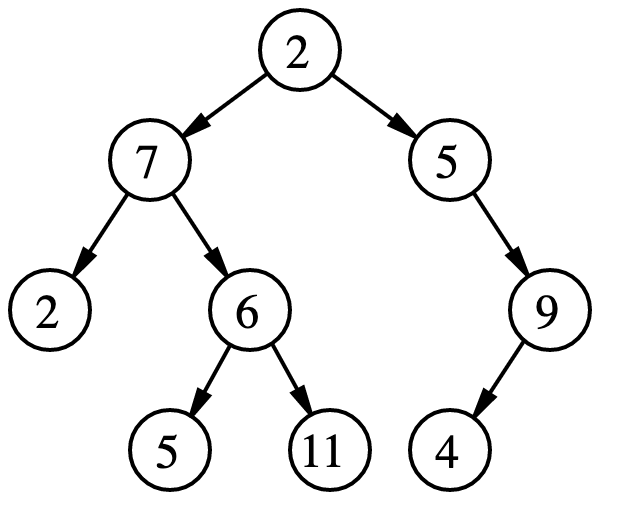There is a certain terminology associated with (binary) trees:

1. depth of a node: is the length of path from the node to the root of the tree.

2. If a node A is in on the path from a node B to root node R, then A is called an ancestor of B and B a descendant of A.

3. The height of a node is the length of the longest path from the node to one of its descendants. The height of a tree is the height of its root node.

4. A node is a leaf it it has no children.

## Operations

A binary tree should support several operations listed below:

1. int size(Node n)
2. int height(Node n)

Both methods can be easily implemented recursively and run at O(n) time.

## Traversal Algorithm

There are 2 types of traversal algorithms for trees, namely breadth-first traversal and depth-first traversal. Within depth-first traversal algorithms, it can be further divided into 3 different methods: pre-order, in-order, and post-order traversal.

#### Pre-order Tree Traversal

In this traversal algorithm, the root of the tree is visited first and then the sub trees rooted at its children are traversed recursively.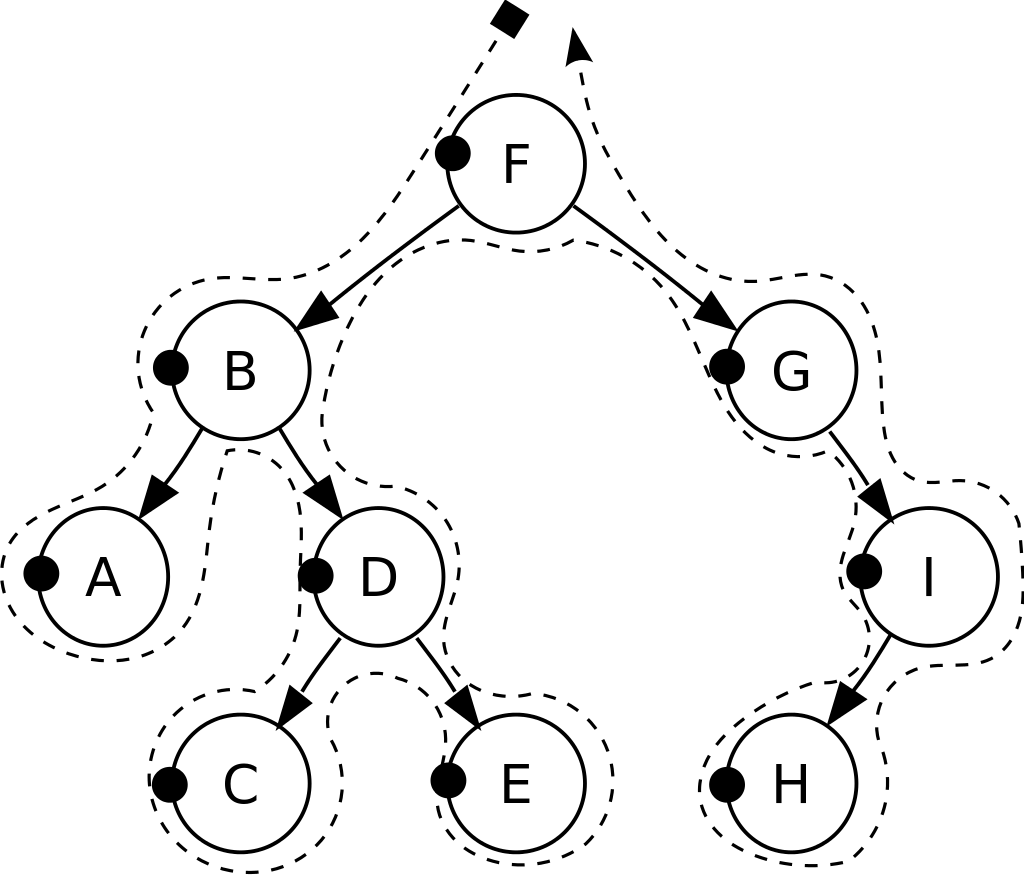With the example in the image above, the output result would be F B A D C E G I H.

#### In-order Tree Traversal

In this traversal algorithm, the tree is visited from left to right. That is, for every position p, the in-order traversal visits p:

• after all the positions in the left sub-tree of p
• before all the positions in the right sub-tree of p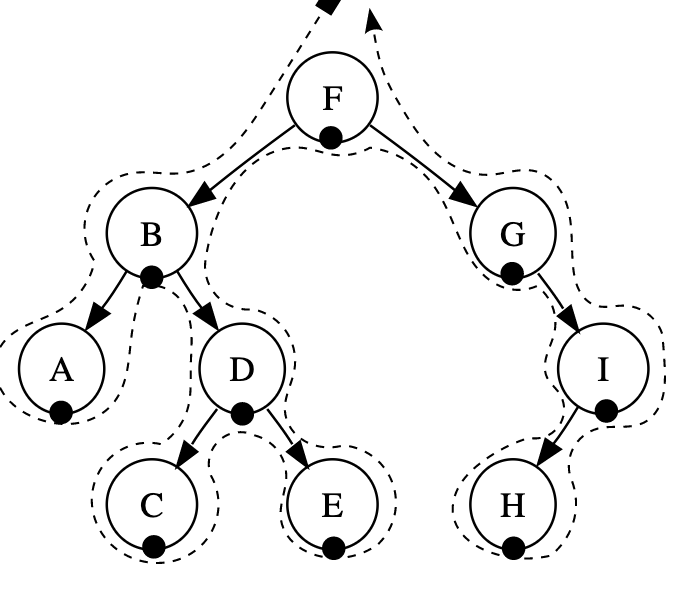With the example in the image above, the output result would be A B C D E F G H I

#### Post-order Tree Traversal

In this traversal algorithm, it recursively traverses the children of the root first and then visit the root.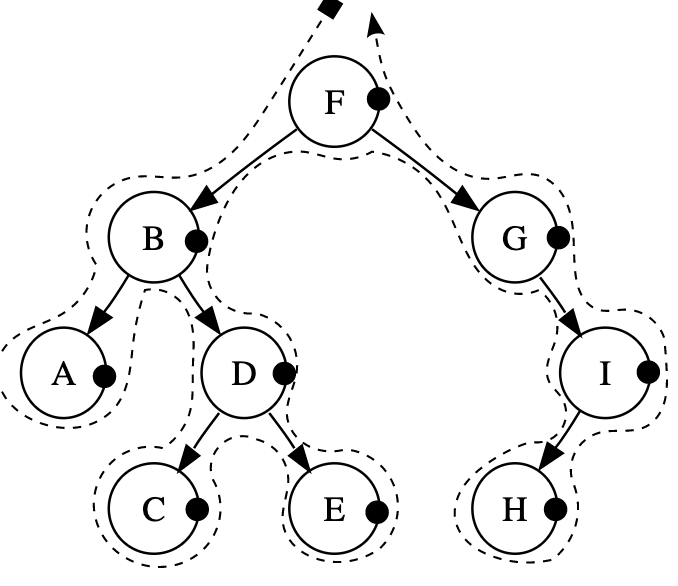With the example in the image above, the output result would be A C E D B H I G F.

In this traversal algorithm, the positions at the same depth d before it visits the positions at depth d+1.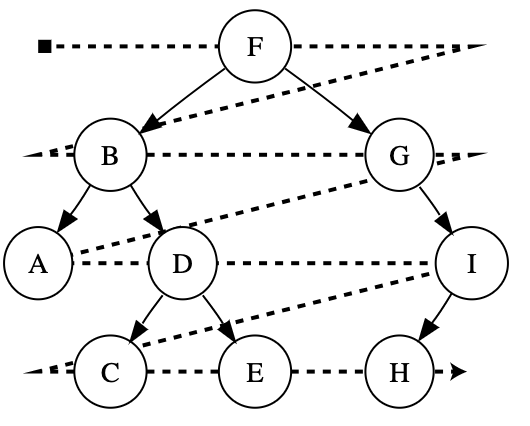To achieve this, we utilise a FIFO queue to give us the ability to traverse in the order in which we visit the nodes.

With the example in the image above, the output result would be F B G A D I C E H.

## Binary Search Tree

A binary search tree is a binary tree T with each position p storing a key-value pair (k,v) such that

• keys stored in the left subtree of p are less than k
• keys stored in the right subtree of p are greater than k

An graphical example of a BST:In a BST, an in-order traversal will visit the keys in ascending order; in this case it’ll be A B C D E F G H I.

## Implementing BST

You can choose to implement the BST with an array or a linked-list-like structure. Here is a link that introduces the implementation with an array. In this post I introduce the linked-list implementation, and therefore there’s the struct node that we need to define first.

The node should store the key value of any type. Note that the key type has to support the operator larger than (>), smaller than(<) and equality (==) for this to work. Therefore if you want to use a custom made struct or class instance, you need to implement operator overloading.

You also need to have some private variables declared in private for the BST class.

Node<K, V>* root = nullptr;
int count = 0;


Searching is quite straightforward given the definition and properties of a BST. When given a key-value pair, we can start from the root node and compare the key of the visited node with the to-be-inserted key. If the key of the visited node is smaller than the to-be-inserted key, we next visit the right child; if the key of the visited node is larger than the to-be-inserted key, we next visit the left child; finally if the visited node’s key is equal to the key to be inserted, we return the visited node.

Note that even if the search is not successful, we still gain valuable information as we would identify the supposed parent node of the to-be-inserted key. In the following implementation, the function return the supposed parent node of the to-be-inserted key if the search is unsuccessful, and it returns the target node of which key is the same as the to-be-inserted-key.

## Insert

With the implementation of search, insertion becomes a lot easier. When given a key-value pair, we call the search function to find the key.

There are 4 cases that the function needs to process:

1. If the key of the returned node is equal to the given key, it means that the key already exists, and we can replace the value of the node with the new value.

2. If the key of the returned node is larger than the given key, we create a new node, store the inserted key and value in it, and attach it to the left child of the returned node.

3. If the key of the returned node is smaller than the given key, we create a new node, store the inserted key and value in it, and attach it to the right child of the returned node.

4. If the node returned by the search function is nil, it means that there’s nothing in the BST yet. In this case, we just need to create a new node, store the necessary values in it and point the root node pointer to this new node.

In the following function implementation, the createNewNode function is just a simple initiation of the struct Node. You can write a custom constructor in the struct declaration and use the new keyword to achieve the same results.

## Remove

Removal is a bit more complicated. Here we need to discuss two cases.

Case 1. the node to be removed has at 0 or 1 child but not 2.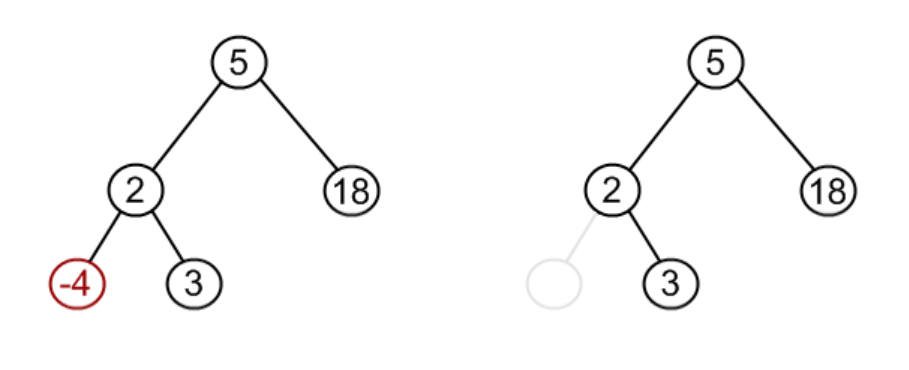If we want to remove the node with key -4 as show in the image, we simply use the node itself to find the parent, identify whether the target node is a left child or the right child, and make it null.

If the target node has one child, it’s similar.

• identify whether the target node has left child or right child.
• identify whether the target node itself is a left child or left child.
• adjust the target node’s parent to point its left or right child pointer to the child node of the target node

We can combine the two cases into one function called splice

Case 2. the node to be removed has 2 children.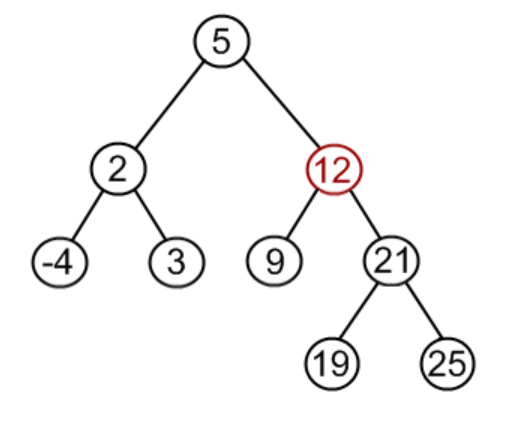In the example above, if we want to remove the node with key 12 (let it be target node T), we need to:

1. find the largest node in its left child subtree (let it be L).
2. swap the keys and values of L and T.
3. splice node L.

It works because L has the key that strictly precedes T’s key (this is based on the definition of a BST). Thus if you swap the keys and values of the aforementioned 2 nodes, all the keys in the right child subtree of T would still be larger than the key of T, and all the keys in the left child subtree of T would be smaller than the key of T

Splicing node L works because node L can at most has 1 child. Being the node with the largest key in a tree, it cannot possibly have a right child.

The findLargest helper function can be easily implemented:

## Runtime Analysis

The search, insert, and remove methods all run in O(h) time where h equals the height of the BST. In the worst case it means O(n) where n is the number of items in the BST. Imagine inserting numbers in an ascending order (1, 2, 3, 4…..n) the items will always be added to the right child. This is not ideal and can be improved with self-balanced BST

## Next…

In the next post I might talk about the self balancing BST that can run the aforementioned methods in $O(\log{n})$ time.

Cheers!